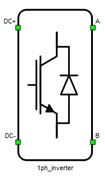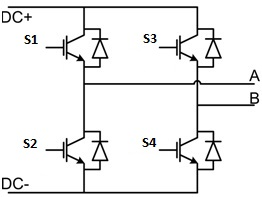# Single Phase Inverter

This section describes single-phase two-level inverter

A block diagram and input parameters for the inverter-rectifier block are given in Table 1.

Table 1. Single-phase two-level inverter/rectifier component in the Schematic Editor core library
component component dialog window component parametersSingle phase inverter• General
• Control (Digital inputs, Internal modulator, Digital input per leg, Model)
• S1 (1..32)
• S2 (1..32)
• S3 (1..32)
• S4(1..32)
• gate_logic (active high, active low)
• Switching enabled (enables usage of external digital signal to enable the switching)
• Sen (digital input selection for PWM enable)
Weight =1

A schematic block diagram of the inverter switching block is given in Figure 1.

Digital inputs, when selected as the Control parameter, enables you to assign gate drive inputs to any of the digital input pins (from 1 to 32). For example, if S1 is assigned to 1, the digital input pin 1 will be routed to the S1 switch gate drive. In addition, the gate_logic parameter is set to either active high (i.e. high-level input voltage VIH turns on the switch), or active low (i.e. low-level input voltage VIL turns on the switch). The gate drive logic depends on your external controller design.Figure 1. A schematic block diagram of a single-phase inverter block diagram with corresponding switch naming

Internal modulator, when selected as the Control parameter, enables you to use the internal PWM modulator for driving the S1, S2, S3, and S4 switches instead of digital input pins. In this configuration, three additional component inputs will be present. The En input is used to enable/disable PWM modulator control, while InA and InB are used as referent signal inputs for the internal PWM modulator. When options for variable frequency or phase are selected, additional component inputs are created: Freq, OffsetA, and OffsetB respectively.

Digital input per leg, when selected as the Control parameter, enables you to assign leg drive input to any of the digital input pins (from 1 to 32). For example, if S1 is assigned to 1, the digital input pin 1 will be routed through the internal dead time module, to the S1 and S2 switch gate drives.

Model, when selected as the Control parameter, enables you to set the IGBTs gate drive signals directly from the signal processing model. The input pin gates appears on the component and requires a vector input of four gate drive signals in the following order: [S1, S2, S3, S4]. When controlled from the model, logic is always set to active high.

Switching enabled, when checked, enables use of an external PWM enabling digital signal.

DTV detection, when enabled, allows DTV detection to be signalized during simulation runtime.

Note: An interactive overview of how you can use DTV detection in your model is available as part of the HIL for Power Electronics course on HIL Academy, as well as in the video Knowledgebase.

## Losses calculation

When the Losses calculation property is enabled, the component will calculate switching and conduction power losses for all switching elements (IGBTs and Diodes or MOSFETs). In the case of MOSFET switching elements, the diode characteristic represents an internal MOSFET body diode. Switching power losses are calculated as a function of current, voltage, and temperature using 3D lookup tables (LUTs). 2D input for losses is also supported. When a 2D losses table is inserted, it assumes only current and temperature dependence. From version 2020.3, conduction power losses can be defined as a function of current and temperature using Vt and Vd lookup tables (the previous option of using Vce, Rce, Vd, and Rd properties are removed). These LUTs can be 1D or 2D tables. If the LUT is a 1D table, forward voltage drop depends only on current, but if the LUT is a 2D table, forward voltage drop dependence on the junction temperature is added. In the MOSFET case under reverse current conduction, a current sharing calculation between MOSFET channel and internal body diode is performed. Import options and an explanation of how to correctly fill all necessary power losses parameters is described in the Importing power losses data section.

Note: An interactive overview of how you can incorporate power loss calculation in your model is available as part of the HIL for Power Electronics course on HIL Academy, as well as in the video Knowledgebase.
Input/output ports for power losses receive/generate vectors of eight elements in the case of the IGBT switch type. The first element (index 0) is the top IGBT in phase A, the second element (index 1) is the upper IGBT's diode in phase A. The next two elements are the phase A bottom IGBT and its diode, respectively. This ordering is valid from the 2019.1 release. The next four elements in the array correspond to phase B in a similar way as phase A. In the case of the MOSFET switch type, input/output ports receive/generate vectors of four elements. The first element (index 0) is the upper MOSFET in phase A and the second element (index 1) is the bottom MOSFET in phase A. The next two elements in the array correspond to phase B in a similar way as for phase A. Available Mask properties are:
• Current values - Switching elements current axis [A]
• Voltage values - Switching elements voltage axis [V]
• Temp values - Switching elements temperature axis [°C]
• Vce - IGBT collector emitter saturation voltage [V] - discontinued from the 2020.3 release
• Rce - IGBT on state slope resistance [Ohm] - discontinued from the 2020.3 release
• Vd - IGBT Diode voltage drop [V] - discontinued from the 2020.3 release
• Rd - IGBT Diode slope resistance [Ohm] - discontinued from the 2020.3 release
• Vt table - Switch forward voltage drop, f(I,T) [V]
• Vd table - Diode forward voltage drop, f(I,T) [V]
• Et on table - Switch switching ON losses, output energy, f(I, V, T) [J]
• Et off table - Switch switching OFF losses, output energy, f(I, V, T) [J]
• Ed off table - Diode switching OFF losses, output energy, f(I, V, T) [J]
Note: Automatic initialization of the Vt table and Vd table properties will be performed based on the discontinued Voltage and Resistance properties (Vce, Rce, Vd, Rd).
Note: Elements ordering for Power losses IGBT Leg terminals in the 2018.4 release was [S_bot_igbt, S_bot_diode, S_top_igbt, S_top_diode] and from the 2019.1 release is changed to [S_top_igbt, S_top_diode, S_bot_igbt, S_bot_diode]. These changes also influence the signal ordering for this component.

## Temperatures calculation

When the Temperatures calculation property is enabled, the component will calculate the combined power losses (P_loss) and junction temperatures (T_junctions) for all switching elements (IGBTs and diodes). Combined power losses represent the sum of the calculated switching and conduction losses transferred through an internally generated Thermal network component. The internally generated Thermal network component also calculates junction temperatures from power losses, input cases temperatures, and provided thermal model parameters. Input/output ports for temperatures calculation are vectors of eight elements and they are indexed in the same way as explained in the Losses calculation section.

Note: An interactive overview of how you can incorporate temperature calculation in your model is available as part of the HIL for Power Electronics course on HIL Academy, as well as in the video Knowledgebase.
• Thermal networks type - Defines type of internal thermal network
• Rth switch - List of thermal resistance for the IGBT switch
• Tth switch / Cth switch - List of thermal time constants or thermal capacitances for the IGBT switch
• Rth diode - List of thermal resistance for diode
• Tth diode / Cth diode - List of thermal time constants or thermal capacitances for diode
• Calculations execution rate - Execution rate in [s] for the losses and temperatures calculation logic

## PESB Optimization

The PESB Optimization option is available in certain converter models. When PESB Optimization is enabled, all converter's short circuit state space modes will be merged and treated as the same state space mode. For example, if one converter leg within the three phase converter is short circuited and PESB Optimization is enabled, all of the legs within the three phase converter will also be short circuited. This simplification for short circuit modeling can save a significant amount of matrix memory.

## Digital Alias

If a converter is controlled by digital inputs, an alias for every digital input used by the converter will be created. Digital input aliases will be available under the Digital inputs list alongside existing Digital input signals. The alias will be shown as Converter_name.Switch_name, where Converter_name is name of the converter component and Switch_name is name of the controllable switch in the converter.